Ask your WordPress questions! Pay money and get answers fast!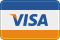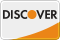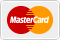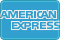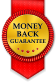# looking for a good way to query posts on a list of specific days. tryi WordPress

• SOLVED

looking for a good way to query posts on a list of specific days. trying to create a total count for each user based on a list of dates. I get passed the following array with details.

id = user id
count = total number of dates
dates = array of dates.

1. The maximum number of dates would be 31 as days don't repeat.
2. I can't do a date range as I need to gather specific totals of posts for a user on the list of dates.
3. I could have between 1-20 users, with a maximum of 31 dates each.
4. Each date could have 0 to 20 posts.
5. I'm adding the total of these posts on each day together for each user.
6. date format is m/d/y

Example:  => Array

1/27/2018 --> 6 posts
2/7/2018 --> 14 posts
so user id 4 has 20 posts.

```  => Array ( [id] => 4 [count] => 2 [dates] => Array (  => 1/27/2018  => 2/7/2018 ) )  => Array ( [id] => 5 [dates] => Array (  => 1/20/2018  => 1/28/2018  => 2/10/2018 ) ) ```

2018-02-17

Is it possible you can show me your site ?

No I have everything run locally, I'm basically wanting to do a query on each of the dates for each user to find the total posts. I would just like to avoid all sorts of nested loops.

2018-02-17

Hello,
That's code is what you need exactly and we have add it to our demo site and working very good

``` /** * Your requested function */ \$value = array(); \$output = array(); foreach( get_users() as \$user ){ \$dates = array(); \$posts = get_posts( array('author' => \$user->ID, 'posts_per_page' => -1) ); foreach(\$posts as \$p){ \$date = date('m/d/Y', strtotime(\$p->post_date)); if( !in_array(\$date, \$dates) ){ array_push(\$dates, \$date); } } \$value['id'] = \$user->ID; \$value['count'] = count(\$dates); \$value['dates'] = \$dates; array_push(\$output, \$value); } print_r( \$output); ```

This is the output
_______________
``` Array (  => Array ( [id] => 1 [count] => 7 [dates] => Array (  => 02/17/2018  => 1/16/2018  => 12/5/2017  => 11/15/2017  => 10/29/2017  => 10/19/2017  => 09/19/2017 ) )  => Array ( [id] => 2 [count] => 5 [dates] => Array (  => 02/17/2018  => 02/16/2018  => 02/15/2018  => 01/15/2018  => 12/19/2017 ) ) ) ```

We can send the demo page contains your code to check it

2018-02-17

Here's one way, if I understand correctly, if you want to use the date query in WP_Query:

We define this helper function:

```/** * Helper function - Number of posts per user * * @uses WP_Query * * @param int \$user_id User ID. Default 0. * @param array \$dates Array of m/d/y dates. Default empty. * @return int \$return Number of found posts. */ function wpq_post_count_per_user( \$user_id = 0, \$dates = [] ) { \$args = [ 'fields' => 'ids', 'author' => (int) \$user_id, 'nopaging' => true, 'ignore_sticky_posts' => true, 'date_query' => [ 'relation' => 'OR', ], ]; foreach( (array) \$dates as \$date ) { \$args['date_query'][] = [ 'year' => date( 'Y', strtotime( \$date ) ), 'month' => date( 'm', strtotime( \$date ) ), 'day' => date( 'j', strtotime( \$date ) ), ]; } \$query = new WP_Query( \$args ); return \$query->found_posts; } ```

Usage example:

```\$input = [ [ 'id' => 1, 'count' => 3, 'dates' => [ '1/20/2018', '1/22/2018', '1/18/2018', ], ], [ 'id' => 2, 'count' => 3, 'dates' => [ '1/28/2018', '1/29/2018', '1/30/2018', ], ], ]; // Number of posts for a given user echo wpq_post_count_per_user( \$input['id'], \$input['dates'] ); ```

Hope it helps.

2018-02-19

```\$date_start = '2018-01-01'; \$date_end = '2018-01-31'; \$res = \$wpdb->get_results( \$wpdb->prepare(" select count(ID) `count`, post_author, DATE(post_date) as day, DATE_FORMAT(post_date, '%c/%e/%Y') nice_day from {\$wpdb->prefix}posts where post_date>=%s and post_date<=%s and post_type='post' and (post_status='publish' OR post_status='private') group by post_author, day order by day, post_author", \$date_start, \$date_end )); \$users = array(); foreach ( \$res as \$row ) { if ( !isset( \$users[ \$row->post_author ] ) ) \$users[ \$row->post_author ] = array( 'id' => \$row->post_author, 'count' => 0, 'dates' => array(), ); \$users[ \$row->post_author ]['count']++; \$users[ \$row->post_author ]['dates'][] = \$row->nice_day; } \$users = array_values( \$users );```